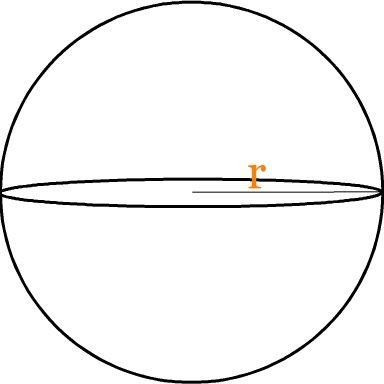# Surface Area of a Sphere Formula

A ball is spherical shaped object. When we see a perfectly symmetrical 3D circular object, then we call it a sphere. A sphere comes without any edges. If we plot two random points on the surface of the sphere then they will be of same distance from center. The line that would pass through the radius of the sphere is the longest line. The area is denoted with respect to square units.The Surface Area of a Sphere Formula is,

$$\begin{array}{l}\large Surface\;Area\;of\;a\;Sphere=4\pi r^{2}\end{array}$$

Where,
r is the radius of the sphere.

### Solved Examples

Question: What will be the surface area of a sphere of radius 7 cm?

Solution:

Given,
r = 7 cm

Surface area of a sphere = 4πr2

= 4

$$\begin{array}{l}\times\end{array}$$
π
$$\begin{array}{l}\times\end{array}$$
(7 cm)2
= 4
$$\begin{array}{l}\times\end{array}$$
π
$$\begin{array}{l}\times\end{array}$$
49 cm2
= 615.44 cm2